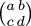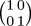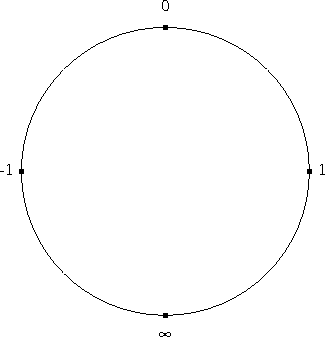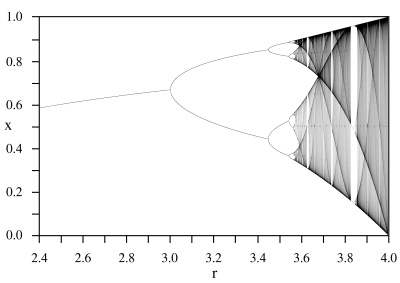# The Universe of Discourse

Sat, 12 Jul 2008

Period three and chaos
In the copious spare time I have around my other major project, I am tinkering with various stuff related to Möbius functions. Like all the best tinkering projects, the Möbius functions are connected to other things, and when you follow the connections you can end up in many faraway places.

A Möbius function is simply a function of the form f : x → (ax + b) / (cx + d) for some constants a, b, c, and d. Möbius functions are of major importance in complex analysis, where they correspond to certain transformations of the Riemann sphere, but I'm mostly looking at the behavior of Möbius functions on the reals, and so restricting a, b, c, and d to be real.

One nice thing about the Möbius functions is that you can identify the Möbius function f : x → (ax + b) / (cx + d) with the matrix, because then composition of Möbius functions is the same as multiplication of the corresponding matrices, and so the inverse of a Möbius function with matrix M is just the function that corresponds to M-1. Determining whether a set of Möbius functions is closed under composition is the same as determining whether the corresponding matrices form a semigroup; you can figure out what happens when you iterate a Möbius function by looking at the eigenvalues of M, and so on.

The matrices are not quite identical with the Möbius functions, because the matrixand the matrix !!{ 2\, 0 \choose 0\,2}!! are the same Möbius function. So you really need to consider the set of matrices modulo the equivalence relation that makes two matrices equivalent if they are the same up to a scalar factor. If you do this you get a group of matrices called the "projective linear group", PGL(2). This takes us off into classical group theory and Lie groups, which I have been intermittently trying to figure out.

You can also consider various subgroups of PGL(2), such as the subgroup that leaves the set {0, 1, ∞, -1} fixed. The reciprocal function x → 1/x is one such; it leaves 1 and -1 fixed and exchanges 0 and ∞.

In general a Möbius function has three degrees of freedom, since you can choose the four constants a, b, c, and d however you like, but one degree of freedom is removed because of the equivalence relation—or, to look at it another way, you get to pick b/a, c/a, and d/a however you like. So in general you can pick any p, q, and r and find the unique Möbius function m with m(0) = p, m(1) = q, m(-1) = r. These then determine m(∞), which turns out to be (4qr - 2p(q+r))/(q + r - 2p) when that is defined. And sometimes even when it isn't.

You may be worrying about the infinities here, but it's really nothing much to worry about. f(∞) is nothing more than !!\lim_{x\rightarrow\infty} f(x)!!.

If (4qr - 2p(q+r))/(q + r - 2p) in the presence of infinities worries you, try a few examples. For instance, consider m : xx+1. This function has p = m(0) = 1, q = m(1) = 2, r = m(-1) = 0. Plugging into the formula, we get m(∞) = -2pq/(q - 2p) = -4 / (2-2) = -4/0 = ∞, which is just right.

The only other thing you have to remember is that +∞ = -∞, because we're really living on the Riemann sphere. Or rather, we're living on the real part of the Riemann sphere, but either way there's only one ∞. We might call this space the "Riemann circle", but I've never heard it called that. And neither has Google, although it did turn up a bulletin board post in which someone else asked the same question in a similar context. There's a picture of it farther down on the right.

Anyway, most choices of p, q, and r in {0, 1, ∞, -1} do not get you permutations of {0, 1, ∞, -1}, because they end up mapping ∞ outside that set. For example, if you take p = 1, q = -1, r = 0, you get m(∞) = -2/3. But obviously the identity function has the desired property, and if you think about the Riemann circle (excuse me, Riemann sphere) you immediately get the rest: any rigid motion of the Riemann sphere is a Möbius function, and some of those motions permute the four points {0, 1, ∞, -1}. In fact, there are eight such functions, because {0, 1, ∞, -1} are at the vertices of a square, so any rigid motion of the Riemann sphere that permutes {0, 1, ∞, -1} must be a rigid motion of that square, and the square has eight symmetries, namely the elements of the group D4:D4 element m(0) m(1) m(∞) m(-1) m(x) = ? M
Identity 0 1 -1 x
 1 0 0 1
Rotate
clockwise
1 -1 0 (x + 1) / (- x + 1)
 1 1 -1 1
Rotate 180° -1 0 1 - (1/x)
 0 -1 1 0
Rotate
counterclockwise
-1 0 1 (x - 1) / (x + 1)
 1 -1 1 1
Reflect
horizontally
0 -1 1 -x
 -1 0 0 1
Reflect
vertically
1 0 -1 1/x
 0 1 1 0
Reflect
diagonally (1)
1 0 -1 (-x + 1) / (x + 1)
 -1 1 1 1
Reflect
diagonally (2)
-1 1 0 (x + 1) / (x - 1)
 1 1 1 -1

Here we have eight functions on the reals which make the group D4 under the operation of composition. For example, if f(x) = (x-1)/(x+1), then f(f(f(f(x)))) = x. Isn't that nice?

Anyway, none of that was what I was really planning to talk about. (You knew that was coming, didn't you?)

What I wanted to discuss was the function f : x → 1 / (1 - x). I found this function because I was considering other permutations of {0, 1, ∞, -1}. The f function takes 0 → 1 → ∞ → 0. (It also takes -1 → 1/2, and so is not one of the functions in the D4 table above.) We say that f has a periodic point of order 3 because f(f(f(x))) = x for some x; in this case at least for x ∈ {0, 1, ∞}.

A function with a periodic point of order three is not something you see every day, and I was somewhat surprised that as simple a function as 1/(1-x) had one. But if you do the algebra and calculate f(f(f(x))) explicitly, you find that you do indeed get x, so every point is a periodic point of order 3, or possibly 1.

Or you can do a simpler calculation: since f is the Möbius function that corresponds to the matrix F = !!{ \hphantom{-}0\, 1 \choose -1\,1}!!, just calculate F3. You get !!{ -1\, \hphantom{-}0 \choose \hphantom{-}0\, -1}!!, which is indeed the identity function.

This also gives you a simple matrix M for which M7 = M, if you happened to be looking for such a thing.

I had noticed a couple of years ago that this 1/(1-x) function had period 3, and then forgot about it. Then I noticed it again a few weeks ago, and a nagging question came into my mind, which is reflected in a note I wrote in my notebook at that point: "WHAT ABOUT SARKOVSKY'S THEOREM?"

Well, what about it? Sharkovskii's theorem (I misspelled it in the notebook) is a delightful generalization of the "Period three implies chaos" theorem of Li and Yorke. It says, among other things, that if a continuous function of the reals has a periodic point of order 3, then it also has a periodic point of order n for all positive integers n. In particular, we can take n=1, so the function f, which has a periodic point of order 3 must also have a fixed point. But it's quite easy to see that f has no fixed point on the reals: Just put f(x) = 1/(1-x) = x and solve for x; there are no real solutions.

So what about Sharkovskii's theorem? Oh, it only applies to continuous functions, and f is not, because f(1) = ∞. So that's all right.

The Sharkovskii thing is excellent. The Sharkovskii ordering of the integers is:

3 < 5 < 7 < 9 < ...
< 6 < 10 < 14 < 18 < ...
< 12 < 20 < 28 < 36 < ...
...
... < 16 < 8 < 4 < 2 < 1.

And the theorem says that if a continuous function of the reals has a periodic point of order n, then it also has a periodic point of order m for all m > n in the Sharkovskii ordering. So if the function has a periodic point of order 2, it must also have a fixed point; if it has a periodic point of order 4, it must also have a periodic point of order 2; if it has a periodic point of order 17, it must also have periodic points of all even orders and all odd orders greater than 17, and so on.

The 1/(1-x) function led me to read more about Sharkovskii's theorem and its predecessor, the "period three implies chaos" theorem. Isn't that a great name for a theorem? And Li and Yorke knew it, because that's what they titled their paper. "Chaos" in this context means the following: say that two values a and b are "scrambled" by f if, for any given d and ε, there is some n for which |fn(a) - fn(b)| > d, and some m for which |fm(a) - fm(b)| < ε. That is, a and b are scrambled if repeated application of f drives a and b far apart, then close together, then far apart again, and so on. Then, if f is a continuous function with a periodic point of order 3, there is some uncountable set S of reals such that f scrambles all distinct pairs of values a and b from S. All that was from memory; I hope it got it more or less correct.

(The Li and Yorke paper also includes an example of a continuous function with a periodic point of order 5 but no periodic point of order 3. It's pretty simple.)

 BuyChaosfrom Bookshop.org(with kickback)(without kickback)
Reading about Sharkovskii's theorem and related matters led me to the web pages of James A. Yorke (of Li and Yorke), and then to the book Chaos: An Introduction to Dynamical Systems that he did with Alligood and Sauer, which is very readable.

I was pleased to finally be studying this material, because it was a very early inspiration to me. When I was about fourteen, my cousin Alex, who is an analytic chemist, came to visit, and told me about period-doubling and chaos in the logistic map. (It was all over the news at the time.) The logistic map is just f : x → λx(1-x) for some constant λ. For small λ, the map has a single fixed point, which increases as λ does. But at a certain critical value of λ (λ=3, actually) the function's behavior changes, and it suddenly begins to have a periodic point of order 2. As λ increases further, the behavior changes again, and the periodicity changes from order 2 to order 4. As λ increases, this happens again and again, with the splits occurring at exponentially closer and closer values of λ. Eventually there is a magic value of λ at which the function goes berserk and is chaotic. Chaos continues for a while, and then the function develops a periodic point of order 3, which bifurcates...(The illustration here, which I copied from Wikipedia, uses r instead of λ.)

I was deeply impressed. For some reason I got the idea that I would need to understand partial differential equations to understand the chaos and the logistic map, so I immediately set out on a program to learn what I thought I would need to know. I enrolled in differential equations courses at Columbia University instead of in something more interesting. The partial differential equations turned out to be a sidetrack, but in those days there were no undergraduate courses in iterated dynamic systems.

I am happy to discover that after only twenty-five years I am finally arriving at the destination.

Cousin Alex also told me to carry a notebook and pen with me wherever I went. That was good advice, and it took me rather less time to learn.